# Current Electricity

Current is the rate of flow of charge defined by:

$$I = \frac {\Delta Q}{\Delta T}$$

where $\Delta Q$ is the charge in coulombs, $I$ is the current and $\Delta T$ is the time taken. The coulomb is the unit of charge. One coulomb is the amount of charge that passes a fixed point in one second with a current of 1A. Charge can be measured using an ammeter attached in series to a circuit.

### Potential Difference

Potential difference (pd) or voltage is the work done per coulomb of charge that passes between two points. It can be calculated by:

$$V = \frac{W}{Q}$$

where $V$ is the voltage, $W$ is the energy in joules and $Q$ is the charge in coulombs ($C$).

### Volt

A volt is defined as one joule per coulomb.
$$1V = 1JC^{-1}$$

### Resistance

When current passes through a circuit it encounters resistance to the flow. A component's resistance can be thought as a measure of how difficult it is for current to flow through a component. Ohms Law states that:

$$V = IR$$
where $V$ is voltage in volts, $I$ is current in amperes and $R$ is resistance measured in ohms ($\Omega$).

Ohms law states that, provided the resistance remains constant, the potential difference is directly proportional to the current. For ohmic conductors (conductors which obey Ohms Law), the graph of $IV$ is shown below: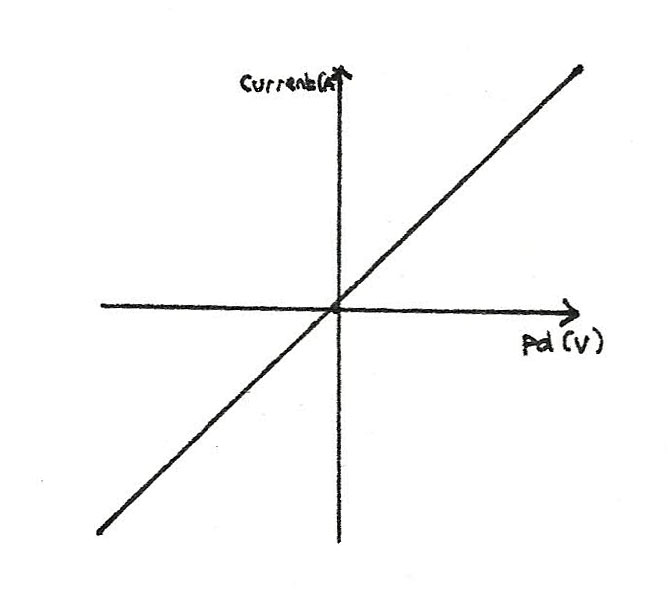## Power

Power ($P$) is defined as the rate of energy transfer. Power is measured in watts (W), where 1 watt is equal to 1 joule per second. The equation power in electrical circuits is:

$$P = VI$$

Where $P$ is the power in watts, $V$ is the voltage and $I$ is current. By combining this equation with $V = IR$, variations can be made:

$$P = \frac{V^2}{R} \\ P = I^{2}R$$

### Worked Example

A heater converts 750J of electrical energy into heat every 10 seconds. What is the power rating of the heater?

\eqalign{ P &= \frac {E}{t} \\ P &= \frac {750}{10} \\ &= 75W}

The total resistance of the heater is $5\Omega$. What current flows through the heater?

\eqalign{ P &= I^{2}R \\ I &= \sqrt{\frac {P}{R}} \\ I &= \sqrt{\frac {75}{5}} I &= 5A}

### Energy

The total energy transferred in a circuit can be found using the following equation:

$$E = VIt$$

## IV Characteristics

This term refers to how voltage and current are proportional. By plotting a graph, the resistance can be calculated by finding the gradient; the shallower the gradient, the greater the resistance. The diagram below shows a circuit arrangement, allowing IV graphs to be plotted.

### Ohmic Conductor

At constant temperature, the current through a metallic conductor is directly proportional to the voltage. This gives a straight line graph passing through the origin.### Filament Lamp

The filament inside of a lamp is a coiled up length of wire which, when a current is passed through it, the temperature increases, increasing the resistance. The resistance increases as the temperature increases.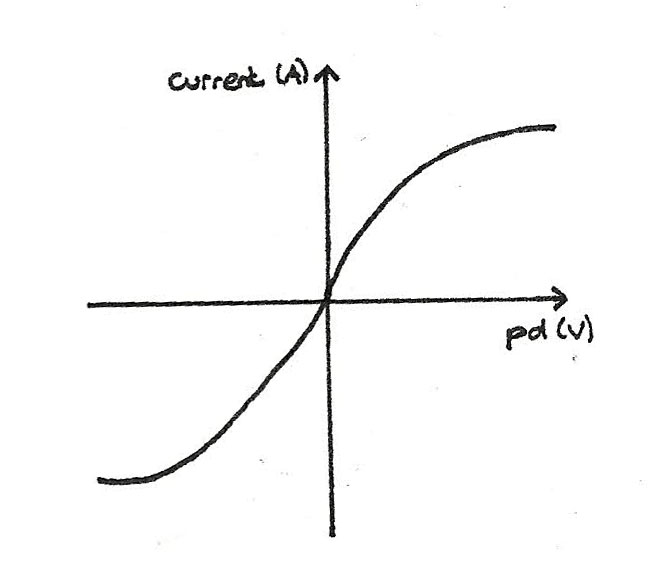### Thermistors

A NTC (negative temperature coefficient) thermistor is a resistor where the resistance decreases as the temperature increases.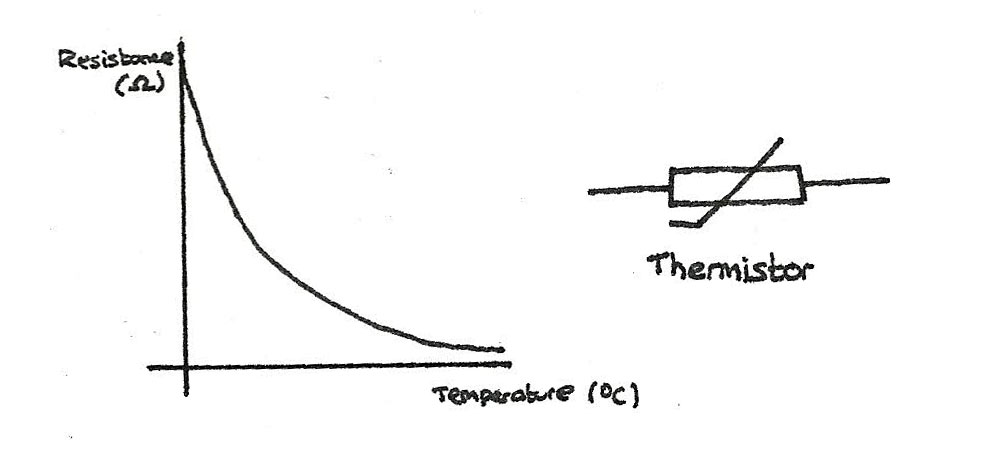### LDR

Light dependant resistors are resistors where the resistance decreases as the light intensity increases.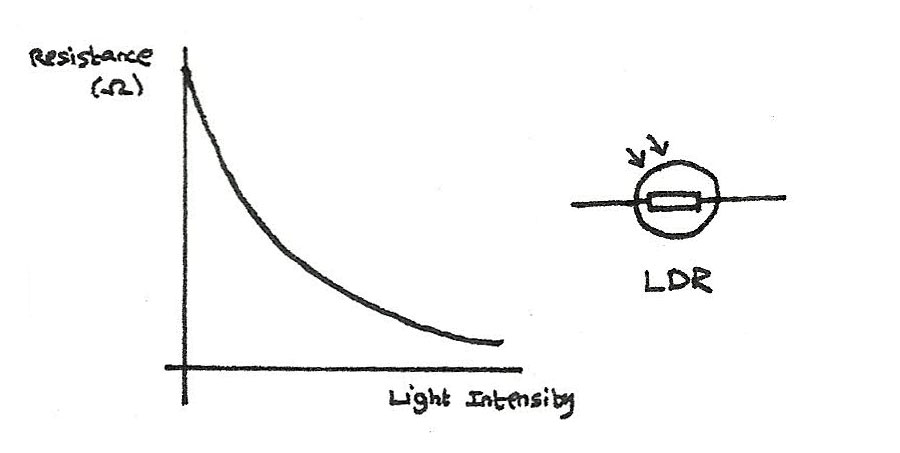### Diodes

Diodes are designed to only allow current to flow in one direction. The forward bias of a diode is the direction in which the current is allowed to flow. Most diodes have a threshold of around 0.6V before they will conduct.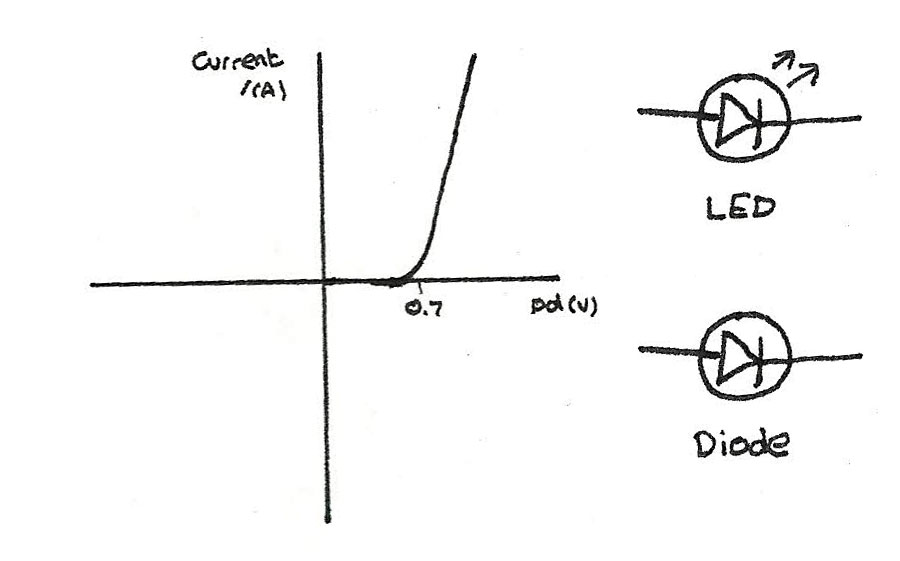## Superconductors

Superconductors are materials that when cooled below a transition temperature, commonly around 10 kelvin (-263 °C), the resistance drops to zero. This allows for:

• Power cables to transmit electricity without any loss of energy
• Strong electromagnets that don't require a constant power source.

The problem with superconductors is that it is difficult and expensive to cool to such low temperatures.